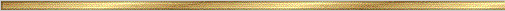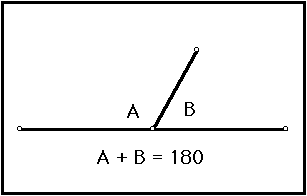# Linear Pair Conjecture### Explanation:

A linear pair of angles is formed when two lines intersect. Two angles are said to be linear if they are adjacent angles formed by two intersecting lines. The measure of a straight angle is 180 degrees, so a linear pair of angles must add up to 180 degrees.

### The precise statement of the conjecture is:

Conjecture (Linear Pairs Conjecture ): Linear pairs of angles add up to 180 degrees.